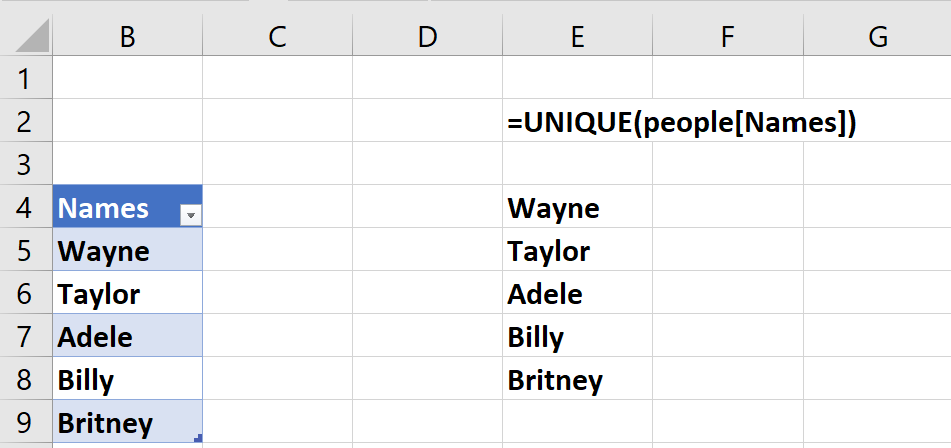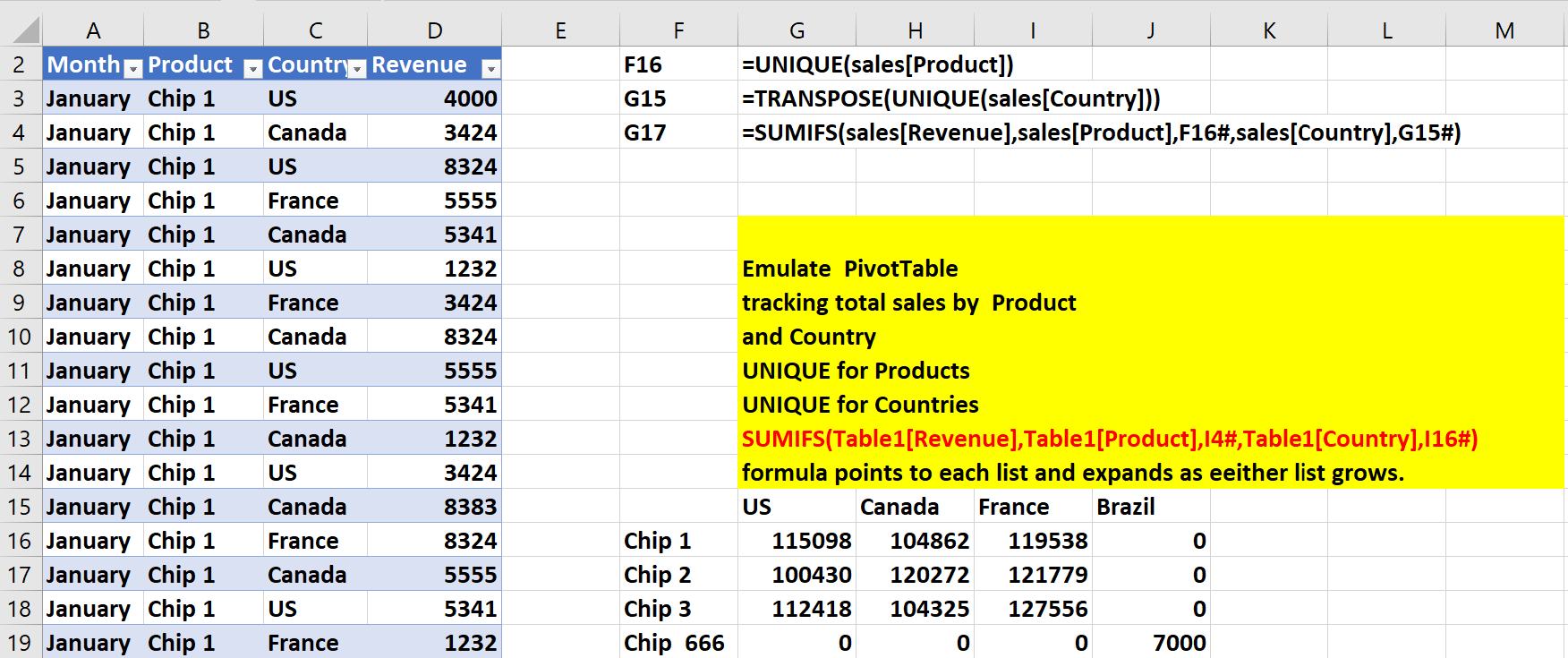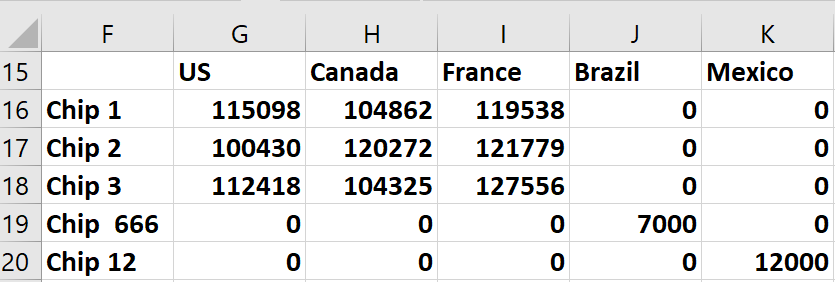# The magical # sign and dynamic array formulasIn our last newsletter, we introduced you to three of Office 365’s amazing dynamic array formulas:

• The UNIQUE function returns a list of unique (based on one or more columns) entries from a spreadsheet range
• The SORT function allows you to bypass the “sort” option on the data tab and sort data via a formula
• The FILTER function allows you to filter data with a formula instead of using the “filter” option on the data tab

We know from our previous articles that if you make the data referred to with these functions into an Excel table, then the functions automatically update to include changes in the original data or new data added to the table. To do this, select the data and use the keystroke combination CONTROL + T.

For example, as shown in Figure 1 and the worksheet UNIQUE of this Excel workbook we made the range B4:B9 a table (named, “people.”) If we add a new name (say, “Rebecca”) to cell B10, then the formula =UNIQUE(people[Names]) in cell E4 will automatically populate cell B9 with Rebecca.

Figure 1: Enter Rebecca in B10, and Rebecca appears in E9.Dynamic arrays were designed so that a # sign placed after a reference to a cell containing a dynamic array formula results in the formula picking up new data that is created when an Excel table is changed.

We’ll now show you how to use the # sign and the UNIQUE dynamic array function to create the equivalent of a Pivot Table. The calculations will automatically update as new data is added with no need to refresh.

Pivot tables without refresh!

The worksheet “Pivot Table” of the Excel workbook (see Figure 2) contains the following data on 208 transactions: month, product, country and revenue. We want to compute total sales of each product in each country, and have our totals automatically update when new data is added.

Figure 2: Emulating a Pivot TableAfter making the cell range A2:D210 a table (named “sales”), the following three formulas perform the needed calculations:

• In cell F16 the formula =UNIQUE(sales[Product]) will return a list of all products
• In cell G15 the formula =TRANSPOSE(UNIQUE(sales[Country])) returns a list of all countries
• Now entering in cell G16 the (AMAZING) formula =SUMIFS(sales[Revenue],sales[Product],F16#,sales[Country],G15#)  computes all the needed totals. You don’t even need to copy the formula! The F16# and G15# portions of the formula ensure that total revenue is computed for each product-country combination.

Amazingly, if new data is entered starting in row 211 revenue for any new product-country combination is totaled. For example, in row 211 of the worksheet “Pivot Table (2),” we entered revenue of 2000 for Chip 12 in Mexico. As Figure 3 shows, our formulas automatically include this data in the calculations.

Figure 3: New data is automatically included in our calculations!Next month we will show you how to create beautiful maps using Filled Maps and Power Maps!